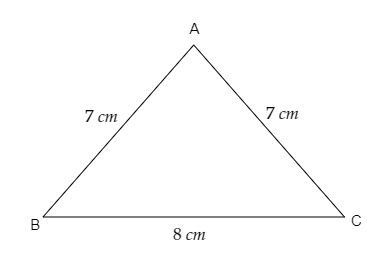# State which of the following are triangles.$\overline{A B}=7 \mathrm{~cm}, \overline{B C}=8 \mathrm{~cm}, \quad \overline{A C}=7 \mathrm{~cm}$

Given:

$\overline{A B}=7 \mathrm{~cm}, \overline{B C}=8 \mathrm{~cm}, \overline{A C}=7 \mathrm{~cm}$

To do:

We have to find if the given measurements form a triangle.

Solution:

In the given triangle,

$\overline{A B}=7 \mathrm{~cm}, \overline{B C}=8 \mathrm{~cm}, \overline{A C}=7 \mathrm{~cm}$

Let us draw a rough figure,As we can see from the figure, $\triangle ABC$ is an isoceles triangle.

Updated on: 10-Oct-2022

25 Views